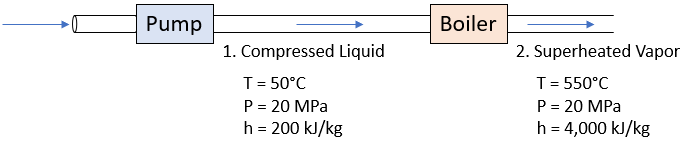## Boiler Pump

The system in the below diagram pumps 60,000 kg of water per hour into a boiler. The pump discharges compressed liquid at 50°C and 20 MPa. The boiler discharges superheated vapor at 550°C and 20 MPa. In the boiler, what is the heat transfer rate to the working fluid?Hint
$$\dot{Q}=\dot{m}(h_2-h_1)$$$where $$\dot{Q}$$ is the heat transfer rate on the working fluid, $$\dot m$$ is the mass flowrate, and $$h$$ is the specific enthalpy. Hint 2 Mass flowrate is measured in kg/sec. An energy balance on the boiler: $$\dot{Q}=\dot{m}(h_2-h_1)$$$
where $$\dot{Q}$$ is the heat transfer rate on the working fluid, $$\dot m$$ is the mass flowrate, and $$h$$ is the specific enthalpy.

Because mass flowrate is measured in kg/sec:
$$\frac{60,000kg}{1hr}\cdot \frac{1hr}{60min}\cdot \frac{1min}{60sec}=\frac{60,000kg}{3,600sec}$$$Thus, $$\dot Q=\frac{60,000kg}{3,600sec}\cdot [(4,000-200)\frac{kJ}{kg}]=(16.67)(3,800)\frac{kJ}{s}$$$
$$\dot Q=63,333\:kW\approx 63\:MW$$\$
63 MW